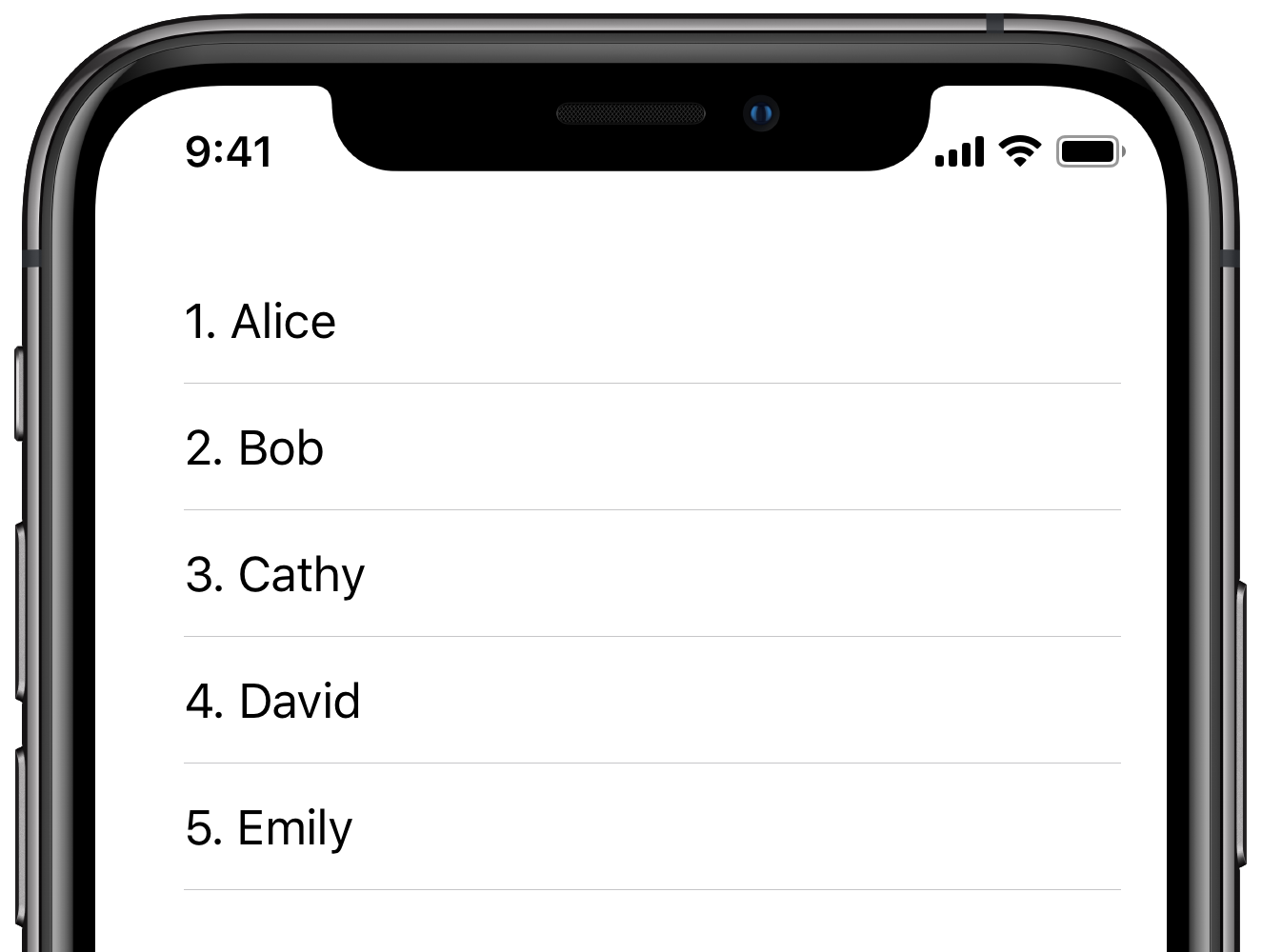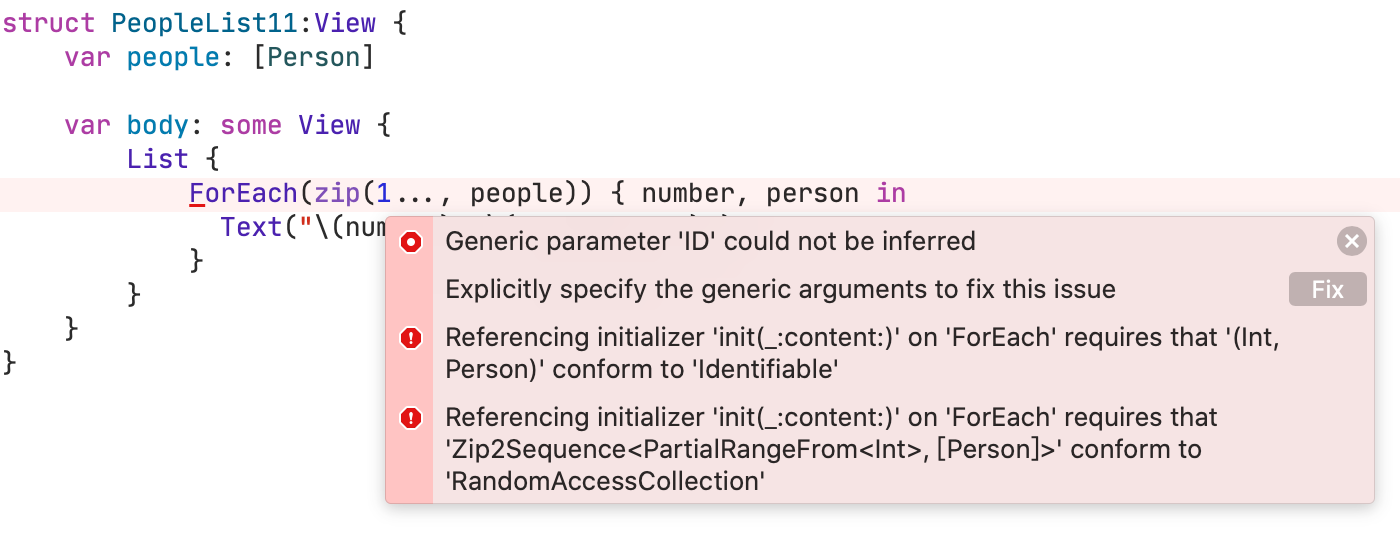# hiccLoghicc log by wccHipo Log

## ForEach中元素的序号``````ForEach(zip(1…, people)) { number, person in
Text("\(number). \(person.name)")
}
``````1. 传给`ForEach`必须是一个`randomaccesscollection`，但是`zip`产出的是一个`Sequence`。 我们通过zip过的序列转换回数组来fix。
2. 序列的元素类型，(Int, Person)不遵循`Identifiable`的协议——这个没法修复，元组不能遵循协议。

``````ForEach(Array(zip(1…, people)), id: \.1.id) { number, person in
Text(“\(number). \(person.name)")
}
``````

## 让代码更易读易用(Clarity at the point of use)

``````extension Sequence {
/// Numbers the elements in `self`, starting with the specified number.
/// - Returns: An array of (Int, Element) pairs.
func numbered(startingAt start: Int = 1) -> [(number: Int, element: Element)] {
Array(zip(start..., self))
}
}
``````

``````ForEach(people.numbered(), id: \.element.id) { number, person in
Text(“\(number). \(person.name)”)
}
``````

## 不使用key path

``````@dynamicMemberLookup
struct Numbered<Element> {
var number: Int
var element: Element

subscript<T>(dynamicMember keyPath: WritableKeyPath<Element, T>) -> T {
get { element[keyPath: keyPath] }
set { element[keyPath: keyPath] = newValue }
}
}
``````

``````extension Sequence {
func numbered(startingAt start: Int = 1) -> [Numbered<Element>] {
zip(start..., self)
.map { Numbered(number: \$0.0, element: \$0.1) }
}
}
``````

``````extension Numbered: Identifiable where Element: Identifiable {
var id: Element.ID { element.id }
}
``````

``````ForEach(people.numbered()) { numberedPerson in
Text(“\(numberedPerson.number). \(numberedPerson.name)”)
}
``````